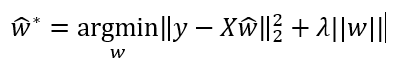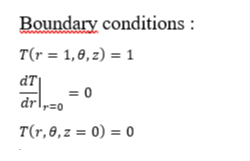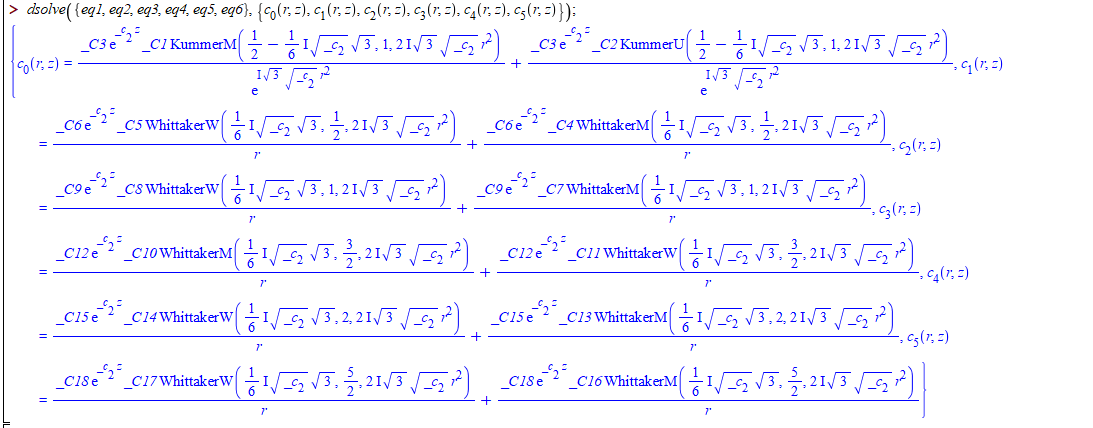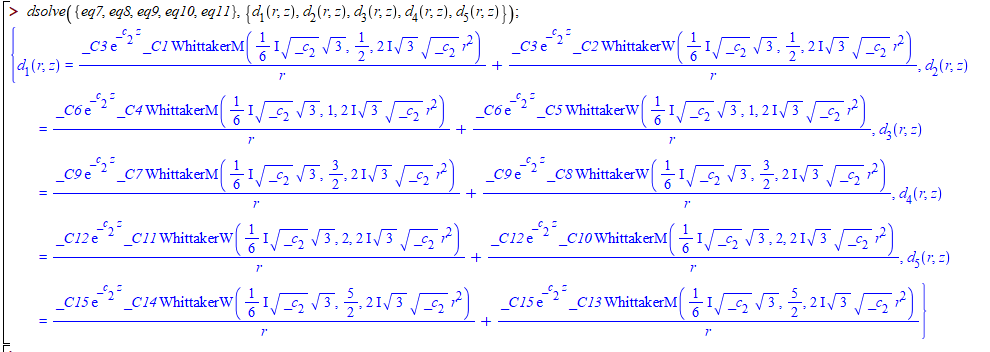## How can we put restrictions of dependency on the s...

Hi to All!

I have an equation including 6 parameters; k_1, k_2, k_3, l_1, l_2, l_3 and a constant a_1. I want to solve this equation for l_1, l_2, and l_3. I get a solution as can be seen in the link but I wonder if there is a solution such that l_j is written in terms of k_j and a_1, j=1,2,3. I mean can be put a restriction on a parameter such that it depends to specific parameters?

Regards,

question.mw

## How do I solve a huge expression for parameters de...

Hi to All! I have a big expression including 10 constant parameters (k_1, k_2, k_3, omega_1, omega_2, omega_3, a_3, b_2, c_0, c_2) to be solved for a_3, b_2, c_0, c_2. I wonder if there is any relation between the constants a_3, b_2, c_0, c_2 not depending on (k_1, k_2, k_3, omega_1, omega_2, omega_3) satisfying this big expression e.g. a_3=2, b_2=c_0/5, c_2 free.

I also tried fsolve but I could not get an answer.

Regards,

question1.mw

## Minimize Matrix equation ...How to slove this equation in Maple (get the optimal w), where y is a vector R(n*1), X is a matrix R(n*m), and w is a vector R(m*1),lambda is a scalar.

## how to simplify the ln(e) in the answer of system ...

How to remove the ln(e) in the fianl answer shown in the picture, ( must be Maple 15), thanks## Numerical evaluation of the Zeta function...

Hello everybody – It looks like there is a bug in the numerical evaluation of (multiple) Zeta function. Take for instance, Zeta(3, 0.5) which is approximately 8.4144. Maple gives approximaterly -96.0033. Is there a bug somewhere?

Thank you

## Maximum value of a function...

Hi Users!

Hope everyone is fine here. I want to find the values of M and L for any functions f(x,y) and y(x) such that

abs(diff(f, y))       for all a ≤ x ≤ b, -∞ < y < ∞ and

abs(diff(y, x, x, x))   for all a ≤ x ≤ b, -∞ < y < ∞

For example for

f:=y-x^(2)+1; 0 ≤ x ≤ 2, -∞ < y < ∞ and

y:=(x+1)^2-.5*exp(x); 0 ≤ x ≤ 2, -∞ < y < ∞

the values of L = 1 and M = 0.5exp(2)

## How to solve a symbolic system of 25 independently...

While solving a math problem, one has to deal with a system of 25 linear equations with a parameter s (Laplace transform variable). i tried formulating the system in a matrix form using GenerateMatrix and inverting the system using solve with LU or QR method but without success. i attach a minimal working environment for the system of equations at hand. Any help or advice is highly appreciated – thank you!

## Construction of a square matrix using 2-dimesional...

Dear Users!
First, I define the following polynomial as:

restart; with(LinearAlgebra); nu := 1/2; M1 := 3; M2 := 3; #(any value of M1 and M2)
for k1 from 0 while k1 <= M1-1 do for k2 from 0 while k2 <= M2-1 do
SGP[M2*k1+k2+1] := simplify(sum((-1)^(k1-i1)*GAMMA(k1+i1+2*nu)*GAMMA(nu+1/2)*x^i1*(sum((-1)^(k2-i2)*GAMMA(k2+i2+2*nu)*GAMMA(nu+1/2)*t^i2/(GAMMA(i2+nu+1/2)*factorial(k2-i2)*factorial(i2)*GAMMA(2*nu)), i2 = 0 .. k2))/(GAMMA(i1+nu+1/2)*factorial(k1-i1)*factorial(i1)*GAMMA(2*nu)), i1 = 0 .. k1))
end do end do;
Lambda := `<,>`(seq(SGP[i], i = 1 .. M1*M2));

then, I want to define a square matrix A of order M1M2 by M1M2 after collocating SGP[k](x,y) at x=(i-1)/(M1-1) and y=(j-1)/(M2-1) for i=1,2,3,...M1, j=1,2,3,...M2.

For example, for M1=2 and M3=3 this matrix A is given as:

A:=Matrix(6, 6, {(1, 1) = SGP(0, 0), (1, 2) = SGP(0, 0), (1, 3) = SGP(0, 0), (1, 4) = SGP(0, 0), (1, 5) = SGP(0, 0), (1, 6) = SGP(0, 0), (2, 1) = SGP(0, 1), (2, 2) = SGP(0, 1), (2, 3) = SGP(0, 1), (2, 4) = SGP(0, 1), (2, 5) = SGP(0, 1), (2, 6) = SGP(0, 1), (3, 1) = SGP(1/2, 0), (3, 2) = SGP(1/2, 0), (3, 3) = SGP(1/2, 0), (3, 4) = SGP(1/2, 0), (3, 5) = SGP(1/2, 0), (3, 6) = SGP(1/2, 0), (4, 1) = SGP(1/2, 1), (4, 2) = SGP(1/2, 1), (4, 3) = SGP(1/2, 1), (4, 4) = SGP(1/2, 1), (4, 5) = SGP(1/2, 1), (4, 6) = SGP(1/2, 1), (5, 1) = SGP(1, 0), (5, 2) = SGP(1, 0), (5, 3) = SGP(1, 0), (5, 4) = SGP(1, 0), (5, 5) = SGP(1, 0), (5, 6) = SGP(1, 0), (6, 1) = SGP(1, 1), (6, 2) = SGP(1, 1), (6, 3) = SGP(1, 1), (6, 4) = SGP(1, 1), (6, 5) = SGP(1, 1), (6, 6) = SGP(1, 1)});

for M1=3 and M=2 the matrix A is given as:

A:=Matrix(6, 6, {(1, 1) = SGP(0, 0), (1, 2) = SGP(0, 0), (1, 3) = SGP(0, 0), (1, 4) = SGP(0, 0), (1, 5) = SGP(0, 0), (1, 6) = SGP(0, 0), (2, 1) = SGP(0, 1/2), (2, 2) = SGP(0, 1/2), (2, 3) = SGP(0, 1/2), (2, 4) = SGP(0, 1/2), (2, 5) = SGP(0, 1/2), (2, 6) = SGP(0, 1/2), (3, 1) = SGP(0, 1), (3, 2) = SGP(0, 1), (3, 3) = SGP(0, 1), (3, 4) = SGP(0, 1), (3, 5) = SGP(0, 1), (3, 6) = SGP(0, 1), (4, 1) = SGP(1, 0), (4, 2) = SGP(1, 0), (4, 3) = SGP(1, 0), (4, 4) = SGP(1, 0), (4, 5) = SGP(1, 0), (4, 6) = SGP(1, 0), (5, 1) = SGP(1, 1/2), (5, 2) = SGP(1, 1/2), (5, 3) = SGP(1, 1/2), (5, 4) = SGP(1, 1/2), (5, 5) = SGP(1, 1/2), (5, 6) = SGP(1, 1/2), (6, 1) = SGP(1, 1), (6, 2) = SGP(1, 1), (6, 3) = SGP(1, 1), (6, 4) = SGP(1, 1), (6, 5) = SGP(1, 1), (6, 6) = SGP(1, 1)});

and for M1=M2=3 the matrix A should be the following form:

A:=Matrix(9, 9, {(1, 1) = SGP(0, 0), (1, 2) = SGP(0, 0), (1, 3) = SGP(0, 0), (1, 4) = SGP(0, 0), (1, 5) = SGP(0, 0), (1, 6) = SGP(0, 0), (1, 7) = SGP(0, 0), (1, 8) = SGP(0, 0), (1, 9) = SGP(0, 0), (2, 1) = SGP(0, 1/2), (2, 2) = SGP(0, 1/2), (2, 3) = SGP(0, 1/2), (2, 4) = SGP(0, 1/2), (2, 5) = SGP(0, 1/2), (2, 6) = SGP(0, 1/2), (2, 7) = SGP(0, 1/2), (2, 8) = SGP(0, 1/2), (2, 9) = SGP(0, 1/2), (3, 1) = SGP(0, 1), (3, 2) = SGP(0, 1), (3, 3) = SGP(0, 1), (3, 4) = SGP(0, 1), (3, 5) = SGP(0, 1), (3, 6) = SGP(0, 1), (3, 7) = SGP(0, 1), (3, 8) = SGP(0, 1), (3, 9) = SGP(0, 1), (4, 1) = SGP(1/2, 0), (4, 2) = SGP(1/2, 0), (4, 3) = SGP(1/2, 0), (4, 4) = SGP(1/2, 0), (4, 5) = SGP(1/2, 0), (4, 6) = SGP(1/2, 0), (4, 7) = SGP(1/2, 0), (4, 8) = SGP(1/2, 0), (4, 9) = SGP(1/2, 0), (5, 1) = SGP(1/2, 1/2), (5, 2) = SGP(1/2, 1/2), (5, 3) = SGP(1/2, 1/2), (5, 4) = SGP(1/2, 1/2), (5, 5) = SGP(1/2, 1/2), (5, 6) = SGP(1/2, 1/2), (5, 7) = SGP(1/2, 1/2), (5, 8) = SGP(1/2, 1/2), (5, 9) = SGP(1/2, 1/2), (6, 1) = SGP(1/2, 1), (6, 2) = SGP(1/2, 1), (6, 3) = SGP(1/2, 1), (6, 4) = SGP(1/2, 1), (6, 5) = SGP(1/2, 1), (6, 6) = SGP(1/2, 1), (6, 7) = SGP(1/2, 1), (6, 8) = SGP(1/2, 1), (6, 9) = SGP(1/2, 1), (7, 1) = SGP(1, 0), (7, 2) = SGP(1, 0), (7, 3) = SGP(1, 0), (7, 4) = SGP(1, 0), (7, 5) = SGP(1, 0), (7, 6) = SGP(1, 0), (7, 7) = SGP(1, 0), (7, 8) = SGP(1, 0), (7, 9) = SGP(1, 0), (8, 1) = SGP(1, 1/2), (8, 2) = SGP(1, 1/2), (8, 3) = SGP(1, 1/2), (8, 4) = SGP(1, 1/2), (8, 5) = SGP(1, 1/2), (8, 6) = SGP(1, 1/2), (8, 7) = SGP(1, 1/2), (8, 8) = SGP(1, 1/2), (8, 9) = SGP(1, 1/2), (9, 1) = SGP(1, 1), (9, 2) = SGP(1, 1), (9, 3) = SGP(1, 1), (9, 4) = SGP(1, 1), (9, 5) = SGP(1, 1), (9, 6) = SGP(1, 1), (9, 7) = SGP(1, 1), (9, 8) = SGP(1, 1), (9, 9) = SGP(1, 1)});

Please help to define general matrix A for any values of M1 and M2. I will be very grateful for you.

## To eliminate some entries of a list that are not ...

Let L=[a1,a2,...,an] be a list of positive real numbers.

My Question:

How to write a procedure to find a minimal interval such as [b,c]

provided that this interval covers entries of L which are close together.

Example:

let L:=[8.1 , 2.03 , 3.5 , 0.05 , 4.1]. Then the output of the procedure is the interval [2.03 , 4.1].

In fact we want to eliminate some entries of L that are not close to other entries of L.

## Using toroidal in PDEchangecoords & defining inver...

Hello everybody,

i am trying to use PDEchangecoords to transform a system of differential equations from Cartesian coordinates to toroidal coordinates. However, when i use a user defined coordinate transform from toroidal to Cartesian, i don't get the initial equations. Please find attached a minimal working environment.

i would highly appreciate your hints and suggestions!

Thank you

Best regards,

F(1)I read the article "ONEOptimal: A Maple Package for Generating One-Dimensional Optimal System of Finite Dimensional Lie Algebra", and also searched out in Maplesoft website, but couldn't found. Does anyone have the package?

## I have a set of boundary conditions that i want t...

`# I solved the differential equation using a fourier series decomposting method. I found with the help of "Dsolve" the different expressions of the fourier constants and now I will apply the boundary conditions to find the expressions of its constants.`

#this my boundary conditions#this my system of equations## Histogram vector, starts from 0...

I have question how start histogram from 0? I have vector with probablity which start from 0.

I generate samples from my probablity:

pr=values of probablity

X:=RandomVariable(ProbablityTable(pr)):

A=Sample(X,1000):

Q=Histogram(A, binwidth=1,range=0..10):

...

I try to use bindwidth=-1 but it's impossible.

How start histogram from 0 for vectors in maple15?

## Cannot find integration...

Cannot find integration proplem_in_maple.mw

 >>>>>>(1)
 >(2)
 >(3)
 >>(4)
 >(5)
 >(6)
 >(7)
 >(8)
 >(9)
 >(10)
 >(11)
 >>(12)
 >(13)
 >(14)
 >(15)
 >(16)
 >## showing an arrow on a curve...

 1 2 3 4 5 6 7Last Page 1 of 47